CBSE Sample Papers for Class 12 Chemistry Paper 1 are part of CBSE Sample Papers for Class 12 Chemistry. Here we have given CBSE Sample Papers for Class 12 Chemistry Paper 1.

## CBSE Sample Papers for Class 12 Chemistry Paper 1

 Board CBSE Class XII Subject Chemistry Sample Paper Set Paper 1 Category CBSE Sample Papers

Students who are going to appear for CBSE Class 12 Examinations are advised to practice the CBSE sample papers given here which is designed as per the latest Syllabus and marking scheme as prescribed by the CBSE is given here. Paper 1 of Solved CBSE Sample Paper for Class 12 Chemistry is given below with free PDF download solutions.

Time Allowed : 3 Hours

Max. Marks : 70

General Instructions

• All questions are compulsory.
• Question number 1 to 5 are very short answer questions and carry 1 mark each.
• Question number 6 to 10 are short answer questions and carry 2 marks each.
• Question number 11 to 22 are also short answer questions and carry 3 marks each.
• Question number 23 is a value based question and carry 4 marks.
• Question number 24 to 26 are long answer questions and carry 5 marks each.
• Use log table, if necessary. Use of calculators is not allowed.

Questions

Question 1.
Why are aquatic species more comfortable in cold water as compared to warm water?

Question 2.
Write IUPAC name of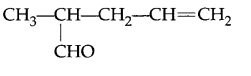Question 3.
Which of the following complex is more stable, write its IUPAC name:
[CO(NH3)6]3+ or [Co(CO)6]3+

Question 4.
Name the method used for the refining of Zirconium metal.

Question 5.
How are deltas formed?

Question 6.
Write two differences between Covalent and Molecular solids.

Question 7.
Calculate ΔG° for the following cell at 298 K.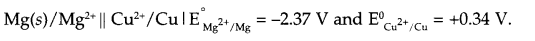Question 8.
An element with density 5.6 g cm 3 exists in bcc lattice with edge length 400 pm. Calculate atomic mass of this element.

Question 9.
Draw the structure of following molecules :
(a) IF4
(b) XeF6

Question 10.
Calculate mass of glucose (molar mass 180 g/mol) to be diluted in 60 g of H2O to lower the freezing point of its solution by 0.93 K (Kf for H2O is 1.86 K kg mol-1)

Question 11.
(a) Which solution is used for the leaching of gold metal in the presence of air in the metallurgy of gold?
(b) Out of C and CO, which is better reducing agent at lower temperature for extraction of iron from its oxide ore.
(c) Write the principle involved in zone refining.

Question 12.
Complete the following reactions:

1. XeF2 + PF5 →
2. H3PO3 $$\underrightarrow { Heat }$$
3. ClO3 + Cl $$\underrightarrow { { H }^{ + } }$$

Question 13.
(a) Chlorobenzene is less soluble in water as compared to chloroethane. Explain.
(b) Which will show faster SN1 reaction and why?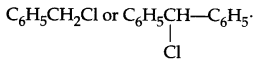(c) Which of the following will be optically active: 2-chlorobutane or 1-chlorobutane?

Question 14.
Explain the mechanism of the following reactions :
(a) C2H5OH $$\xrightarrow [ { Conc.H }_{ 2 }{ SO }_{ 4 } ]{ { 170 }^{ 0 }C }$$ CH2 = CH2
(b) CH3 – OH $$\xrightarrow [ { H }^{ + } ]{ 413K }$$ CH– O – CH3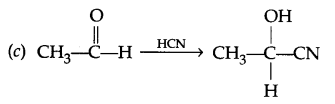Question 15.
(a) Distinguish between lyophilic and lyophobic colloids.
(b) Name the absorbent used for controlling of humidity in operation theatres.

Question 16.
(a) Define the limiting molar conductivity.
(b) State Kohlrausch’s law.
(a) Define corrosion.

Question 17.

1. State Henry’s law.
2. Define azeotropes. What type of azeotropes is formed by positive deviation from Raoult’s law? Give an example.

Question 18.
How would you account for the following?

1. Transition metal exhibits variable oxidation state.
2. Zr(Z = 40) and Hf(Z = 72) have almost identical radii.
3. Transition metals and their compounds act as complexing agents.

OR
Complete the following chemical equations:

1. Cr2O2-7 + Fe2+ + H+
2. CrO2-4 + H+
3. MnO4 + I + H2O →

Question 19.

1. What type of isomerism is exhibited by the complex [Pt(NH3)6] [NiCl6]?
2. Write the formula for potassium hexacyanoferrate (II).
3. Write electronic arrangement of [Co(NH3)6]3+ and discuss its geometry and magnetic behaviour.

Question 20.
Give reason for the following:

1. (CH3)3 P = O exists but (CH3)3 N = O does not.
2. Phosphorus has more negative electron gain enthalpy than nitrogen.
3. H2Se is more acidic than H2O.

Question 21.
Give the structure of A, B and C in the following reactions: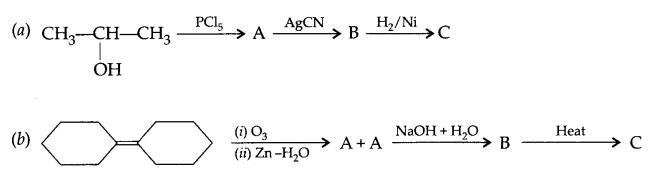Question 22.
Write the name and structure of the monomers of the following polymers
(a) Buna-N
(b) Nylon-2, 6
(c) PVC

Question 23.
Shivani has recently started facing difficulty in visualising in dark. Prema, her best friend wonders why Shivani cannot see properly in the dark, on observation she found that Shivani often carries junk foods in her lunch. Prema has started sharing her lunch full of green vegetables like carrots, tomatoes, coloured fruits, milk and other dairy products. Shivani is now better and has also started taking a balanced diet.

1. Name the disease Shivani is suffering from.
2. Name the Vitamin and its source to cure this disease.
3. What values are shown by Prema?

Question 24.
Account for the following:
(a) pKb of aniline is more than that of methylamine.
(b) Methylamine in water reacts with FeCl3 from hydrated ferric oxide.
(c) Why do primary amines have higher boiling point than tertiary amines?
(d) Complete the following equations:
(i) C6H5N2Cl + C2H5OH →
(ii) C6H5NH2 + (CH3CO)2O →
OR
(a) Give one chemical test to distinguish between:
(i) Methylamine and dimethylamine
(ii) Aniline and Benzyl amine

(b) How will you convert:
(i) Ethanoic acid to Methanamine
(ii) Aniline to p-bromoaniline
(c) Arrange according to increasing basic strength C6H5NH2, C6H5N(CH3)2, (C2H5)2NH, CH3NH2

Question 25.
(a) The rate constant for a first order reaction is 60 s-1. How much time will it take to reduce the initial concentration of the reactant to its l/16th value?
(b) Differentiate between order and molecularity of reaction.
(c) Write unit of rate constant (K) for
(i) First order reaction
(ii) 2nd order reaction
OR

1. The rate of a reaction quadruples when the temperature changes from 290 K to 310 K, calculate the activation energy for this reaction.
2. A reaction is first order wrt. P and wrt. 2nd order wrt. Q, write the differential rate equation for it.
3. Write factors (any two) that affects the rate of a chemical reaction.
4. Define specific rate constant.

Question 26.

1. Phenol is more acidic than cyclohexanol. How?
2. Which is steam volatile, 2-nitrophenol or 4-nitrophenol and why?
3. Explain following name reactions with a suitable chemical reaction.
1. Sandmeyer’s reaction
2. Cannizaro’s reaction

OR
(a) Write the reaction of glucose with HI.
(b) Name the force responsible for stabilisation of secondary structure of protein.
(c) Arrange according to the given instructions in increasing order:
(i) Phenol, Methoxyphenol, 2,4-Dinitrophenol (acidic strength)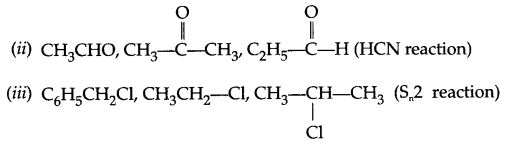In cold water the concentration of dissolved oxygen gas is more and hence respiration for aquatic species will be easier so it will be comfortable as compared to warm water.

2-methyl pent-4-enal

[Co(CO)6]3+ complex is more stable. It is due to synergic bonding between metal and carbonyl group.
IUPAC name → Hexacarbonyl cobalt (III) ion.

van Arkel method

Coagulation of silt present in river water due to electrolytes present in sea water leads to formation of delta.

 Covalent solid Molecular solid (i) High melting point (i) Low melting point (ii) Hard in nature (ii) Soft in nature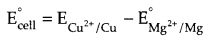= 0.34 – (- 2.37) = 2.71 V
Here change in number of electrons (n) = 2
ΔG° = – nFEcell° = – 2 × 96500 × 2.71 = – 523.03 kJ/mole

Since d = $$\frac { ZM }{ { a }^{ 3 }{ N }_{ A } }$$
From question → bcc arrangement i.e. Z = 2
a = 400 pm = 400 × 10-10 cm = 4 × 10-8 cm
d = 5.6 g/cm3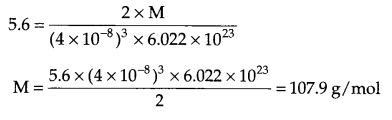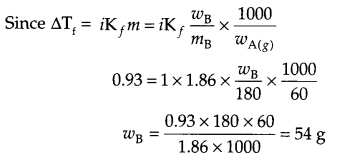i.e. 54 g glucose needs to be added.

1. Aqueous solution of NaCN or KCN is used for leaching.
2. CO at lower temperature is a better reducing agent than C for the extraction of iron from its oxide ore.
3. Impurities are more soluble in molten metal as compared to its solid state.

1. XeF2 + PF5 → [XeF]+ [PF6]
2. H3PO3 $$\underrightarrow { Heat }$$ PH+ H3PO4
3. ClO3 + Cl $$\underrightarrow { { H }^{ + } }$$ Cl2 + H2O

(a) Phenyl group is more hydrophobic as compared to smaller ethyl group, hence chloroethane is more soluble than chlorobenzene.Due to more stable carbocation formation which is there because of more number of resonance structures.
(c) 2-chlorobutane.

(a) H2SO4 + H2O → H3O+ + HSO4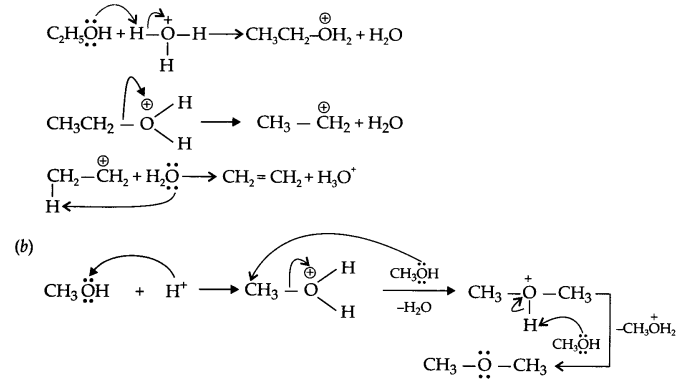(a)

 Lyophilic colloids Lyophobic colloids (i) Strong forces of attraction between dispersed phase and dispersion medium. (i) Weak forces of attraction between dispersed phase and dispersion medium. (ii) Reversible sol once separated can be reformed. (ii) Irreversible sols once separated cannot be reformed. (iii) These are stable in nature. (iii) These are unstable and can be easily precipitate. (iv) Prepared directly on mixing dispersed phase particles with dispersion medium. For example, gum, starch, etc. (iv) Special methods are required for preparation. For example, metal sulphides.

(b) Silica gel

1. Limiting molar conductivity : The value of molar conductivity when the concentration of electrolytes approaches zero is known as limiting molar conductivity or molar conductivity at infinite dilution. It is the maximum possible molar conductivity for any electrolyte. Represented by Δ°m.
2. Kohlrausch’s law : It states that the limiting molar conductivity of on electrolyte is the sum of the individual contribution of the anion and cation of the electrolyte, e.g.
Δ°m(CH3COOH) = λ°CH3COO + λ°H+
3. Corrosion : The tarnishing of upper layer of any active metal due to environmental factors such as water vapours, CO2, O2, etc. in known as corrosion.
For example-rusting of iron, blackening of silver, deposition of greenish layer on copper etc.

(a) Henry’s law : It states that the partial pressure (p) of a gas in vapour phase is proportional to the mol fraction of the gas (x) in the solution. It is expressed as
p = kHx
where kH is known as Henry’s law constant and x is mol fraction.

(b) Azeotropes : It is a binary mixture, having same composition in liquid state as well as in vapour phase and boils at constant temperature like a pure liquid. If there is positive deviation, then minimum boiling azeotrope will form. In case of negative deviations, maximum boiling azeotrope will form.
Examples 95.5% Ethanol and 4.5% H2O by volume.

1. Transition metals exhibits variable oxidation state due to comparable energies of (n – 1)d and ns orbitals, so electrons from both can form bond.
2. Due to lanthanoid contraction.
3. Transition metals acts as complexing agent due to vacant d-orbitals and high charge density on metal ion.

OR

1. Cr2O2-7 + 6Fe2+ + 14H+ → 2Cr3+ + 6Fe3+ + 7H2O
2. CrO2-4 + H+ → Cr2O2-7
3. 2MnO4 + 10 I + 8H2O → 2Mn2+ + 5I2 + 16 OH

1. Co-ordination isomerism is exhibited by the complex [Pt(NH3)6] [NiCl6]
2. K4[Fe(CN)6]
3. Electronic arrangement for [Co(NH3)6]3+ according to VBT.
Co → 3d74s2
Co3+ → 3d64s0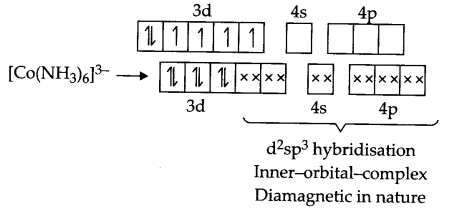1. Due to lack of d-orbital in N, dπ-pπ bonding in not possible, hence formation of (CH3)3 N = O is not possible.
2. Due to small size and high electron density in valence shell of N, its electron gain enthalpy is less than phosphorous.
3. Due to longer H-Se bond than H-O bond H2Se is more acidic.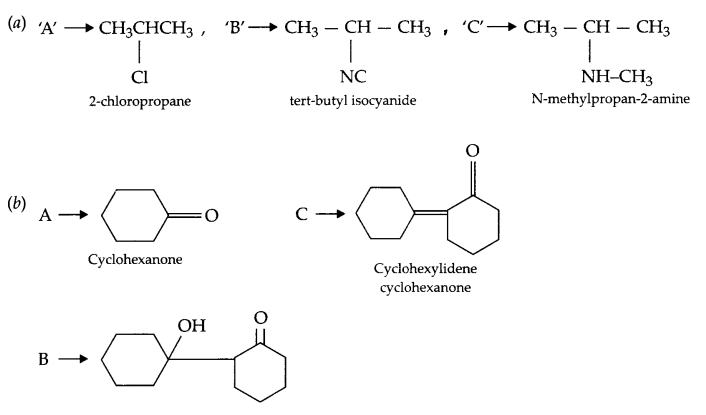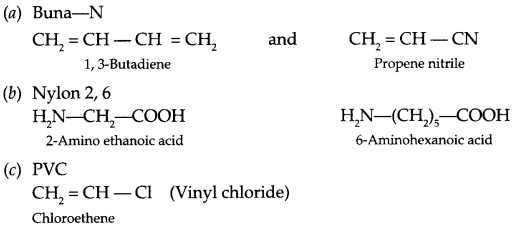1. Night blindness
2. Vitamin-A, Source – Carrot, cod liver oil, butter, milk etc.
3. Care for friend, knowledge of science, sincerity in her effort.

(a) Aniline is a weaker base than methylamine due to positive charge on nitrogen, which is there because of resonance. Hence its pKb value is more than methylamine.
(b) Methyl amine gives OH- ions in water, hence FeCl3 get hydrolysed to give hydrated ferric oxide.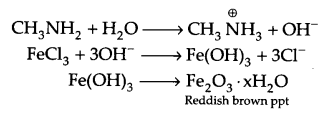(c) There is intermolecular hydrogen bonding in primary amines, which is not possible in tertiary amines. Hence primary amines have higher boiling point.OR
(a) (i)

 Reagent Aniline Benxylamine CHCl3 + KOH Heat Offensive Smell appears No reaction

(ii)

 Reagent Aniline Benxylamine Br water White ppt forms No ppt NaNO2 + HCl, 00 – 50 C Add phenol Orange-red dye form No such dye formation

(b) (i) Ethanoic acid to methanamine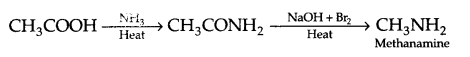(ii) Aniline to p-bromo aniline(c) C6H5NH2 < C6H5N(CH3)2 < CH3NH2 < (C2H5)2NH(b) Difference between order and molecularity:

 Order Molecularity 1. It is experimentally determined with help of rate law equation. 1. It is calculated from balanced chemical reaction or law of mass action equation. 2. It may be positive, negative, zero or fraction. 2. It is always a positive whole number only and cannot be zero or fractional. 3. Applicable for all type of reactions i.e., elementary as well as complex reactions. 3. Applicable only for elementary reactions.

(c) (i) Second-1
(ii) mol-1 L s-1
OR

1. from Arrhenius equation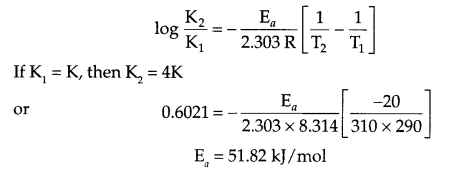2. rate = K[P]1 [Q]2
3. Concentration, pressure, temperature, catalyst
4. It is the rate of reaction when concentration of all the reactants is unity.

1. Since phenoxide ion is resonance stablised, hence phenol is more acidic than cyclohexanol.
2. 2-nitrophenol is steam volatile due to intramolecular hydrogen bonding whereas 4-nitrophenol undergoes intermolecular hydrogen bonding and thus connected to each other to become less volatile.
1. Sandmeyer’s reaction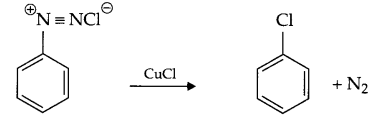2. Cannizaro’s reaction
HCHO $$\underrightarrow { NaOH(Conc.) }$$ CH3OH + HCOONa

OR(b) Intermolecular and intramolecular hydrogen bonding, coloumbic interactions, disulphide